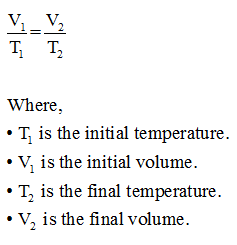# A 3.8 L sample of gas at 1.0 atm and 20 degree C is heated to 83 degree C . calculate the gass volume in liters at higher temperature if the presure remain constant?

Question
2 views

A 3.8 L sample of gas at 1.0 atm and 20 degree C is heated to 83 degree C . calculate the gass volume in liters at higher temperature if the presure remain constant?

check_circle

Step 1

Charles's law states that at a constant pressure, the volume of an ideal gas is directly proportional to the temperature of that ideal gas. The relation between volume and temperature can be represented as,...

### Want to see the full answer?

See Solution

#### Want to see this answer and more?

Solutions are written by subject experts who are available 24/7. Questions are typically answered within 1 hour.*

See Solution
*Response times may vary by subject and question.
Tagged in

### Chemistry